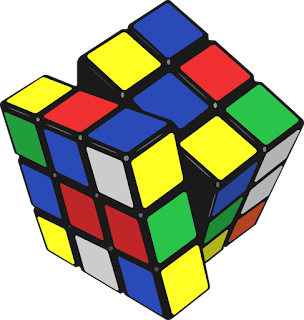## Algebraic Expressions and Identities

### (NCERT Ex 9.2 Answers)

Q1: Find the product of following pair of monomials.

(i) 4 , 7p

Answer:

4   ☓   7p = 4  ☓ 7   ☓ p = 28p

(ii) -4p , 7p

Answer:

-4p   ☓   7p = -4 ☓ p ☓ 7  ☓ p = (-4☓7)☓ (p☓ p) = -28p2

(iii) 4p , 7pq

Answer:

-4p  &#9747 7p = -4 ☓ p ☓7  ☓ p  ☓ q = (-4☓ 7)☓ (p☓ p☓ q) = -28p2q

(iv) 4p3 , -3p

Answer:

4p3  ☓  -3p = 4  ☓(-3)   ☓ p ☓ p☓ p☓ p = -12p4

(v) 4p , 0

Answer:

4p ☓ 0 = 4☓ p☓ 0 = 0

Q2: Find the areas of rectangles with the following pairs of monomials as their lengths
and breadths respectively.

(p , q);(10m , 5n);(20x2 , 5y2);(4x , 3x2);(3mn ,4np)

Answer:

We know that,

Area of rectangle = Length ☓ Breadth

Area of 1st rectangle = p ☓ q = pq

Area of 2nd rectangle = 10m ☓ 5n = 10☓ 5 ☓ m ☓ n = 50mn

Area of 3rd rectangle = 20x2 ☓ 5y2 = 20☓ 5 ☓ x2 ☓ y2 = 100x2y2

Area of 4th rectangle = 4x ☓ 3x2 = 4☓3 ☓ x ☓ x2 = 12x3

Area of 5th rectangle = 3mn ☓4np = 3☓ 4 ☓ m ☓ n ☓ n ☓ p = 12mn2p

Q3: Complete the table of products.

 First Monomial (⇨) Second Monomial(⇩) 2x -5y 3x2 -4xy 7x2y -9x2y2

 2x 4x2 ... ... ... ... ... -5y ... ... -15x2y ... ... ... 3x2 ... ... ... ... ... ... -4xy ... ... ... ... ... ... 7x2y ... ... ... ... ... ... -9x2y2 ... ... ... ... ... ...

Answer:

The table can be completed as follows.

 First Monomial (⇨) Second Monomial(⇩) 2x -5y 3x2 -4xy 7x2y -9x2y2 2x 4x2 -10xy 6x3 -8x2y 14x3y -18x3y2 -5y -10xy 25y2 -15x2y 20xy2 -35x2y2 45x2y3 3x2 6x3 -15x2y 9x4 -12x3y 21x4y -27x4y2 -4xy -8x2y 20xy2 -12x3y 16x2y2 -28x3y2 36x3y3 7x2y 14x3y -35x2y2 21x4y -28x3y2 49x4y2 -63x4y3 -9x2y2 -18x3y2 45x2y3 -27x4y2 36x3y3 -63x4y3 81x4y4

Q4: Obtain the volume of rectangular boxes with the following length,breadth
and height respectivly.

(i) 5a , 3a2 , 7a4

(ii) 2p , 4q , 8r

(iii) xy , 2x2y , 2xy2

(iv) a , 2b , 3c

Answer:

We know that,

Volume = Length ☓ Breadth ☓ Height

(i) Volume= 5a ☓ 3a2☓ 7a4= 5☓ 3☓ 7☓a☓ a2☓a4 = 105a7

(ii) Volume=2p☓ 4q ☓ 8r= 2☓ 4☓ 8☓ p☓ q☓ r = 64pqr

(iii) Volume=xy ☓ 2x2y☓ 2xy2= 2☓2☓ xy☓ x2y☓ xy2 = 4x4y4

(iv) Volume= a ☓ 2b ☓ 3c= 2☓ 3☓ a☓ b☓ c = 6abc

Q5: Obtain the product of

(i) xy , yz , zx

Answer:

xy ☓ yz ☓ zx = x2y2z2

(ii) a , -a2 , a3

Answer:

a☓ -a2☓ a3= -a1 + 2 + 3 = -a6

(iii) 2 , 4y , 8y2 , 16y3

Answer:

2☓ 4y☓ 8y2☓16y3= 2☓ 4☓ 8☓ 16☓y ☓y2☓y3 = 1024y6

(iv) a , 2b , 3c , 6abc

Answer:

a☓ 2b☓ 3c☓ 6abc= 2☓ 3☓ 6☓ a☓ b ☓ c ☓ abc = 36a2b2c2

(v) m , -mn , mnp

Answer:

m ☓ (-mn)☓ mnp= -m3n2p#### Post a Comment

We love to hear your thoughts about this post!